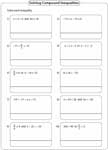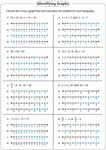Compound Inequalities Worksheets

Each section (except the first section) contains three levels of compound inequalities worksheets based on either solving or graphing or both. The levels are classified based on the number of steps that required solving compound inequalities.

Graphing Compound Inequalities: Basic

No solving required. Graph each basic compound inequality in a number line at the correct interval.Easy:

Moderate:

Solving Compound Inequalities

Solve each compound inequality and find the solutions. Both 'and' and 'or' cases are included.One-step:

Two-step:

Multi-step:

Solving and Graphing Compound Inequalities

Solve each compound inequality and graph the solutions using the number line.One-step:

Two-step:

Multi-step:

Multiple Choices: Standard

Each worksheet contains 10 multiple choices (only eight in multi-step) based on identifying correct solutions for the compound inequalities.One-step:

Two-step:

Multi-step:

Multiple Choices: Graphical Solution

Choose the correct graph that best describes the solution for the compound inequality.One-step:

Two-step:

Multi-step:

Multiple Choices: Interval Notation

Identify the correct solution interval for each compound inequality.One-step:

Two-step:

Multi-step:

Stock up your library with the complete set of worksheets on compound inequalities.

Related Worksheets